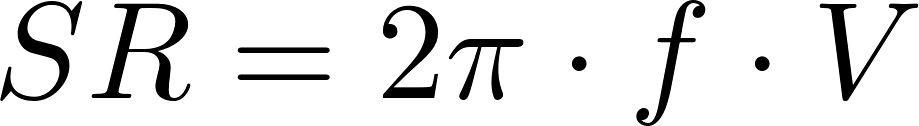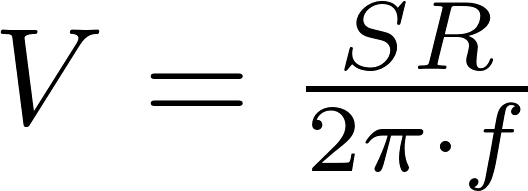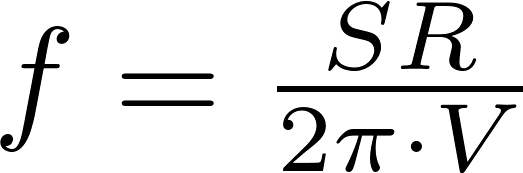# Slew rate

The slew rate is the change of voltage per unit of time. the unit of slew rate is volts per second (V/s).

### FormulasSR is the symbol for slew rate and is measured in volts per second (V/s).
V is the symbol for voltage and is measured in volt (V).
f is the symbol for frequency and is measured in hertz (Hz).

### Calculator

Enter two values to calculate the third.